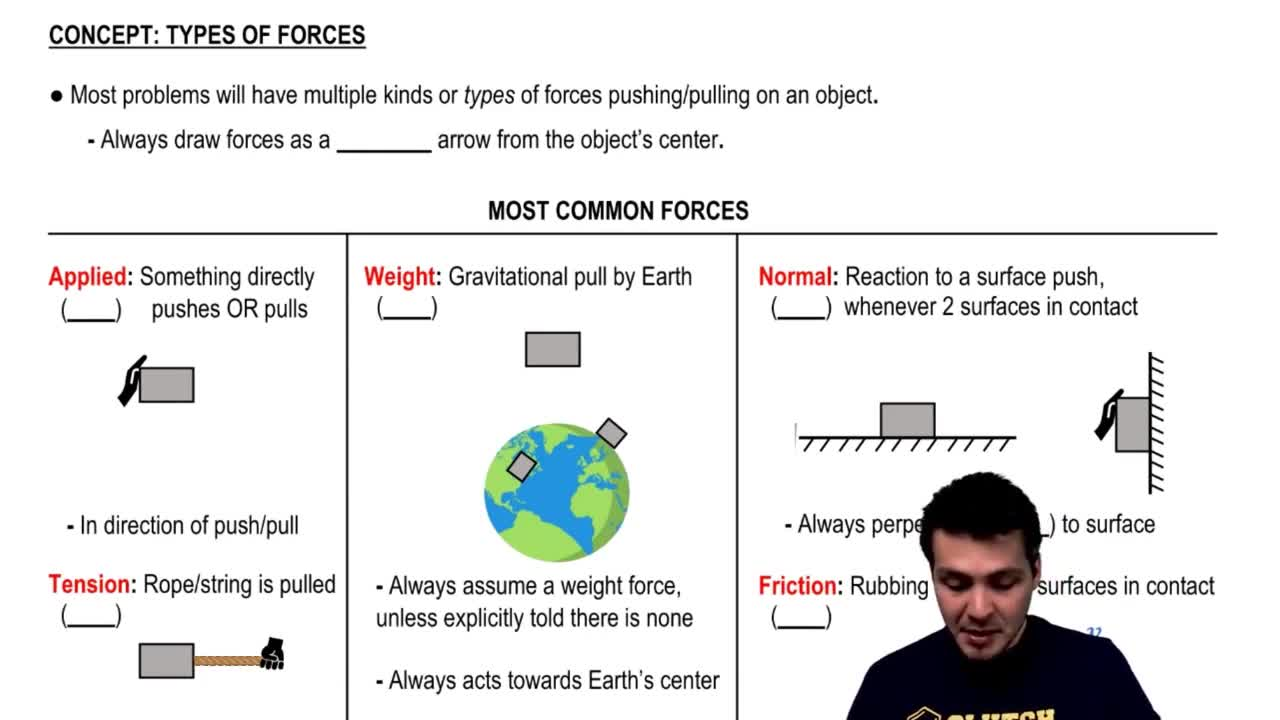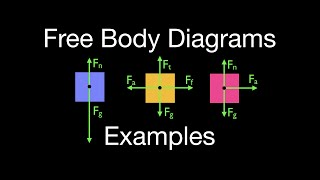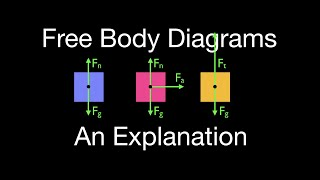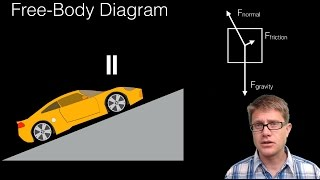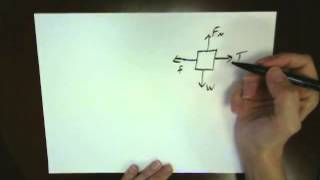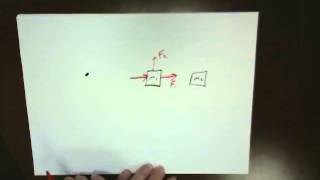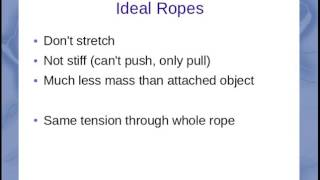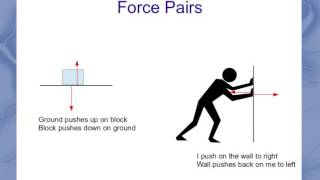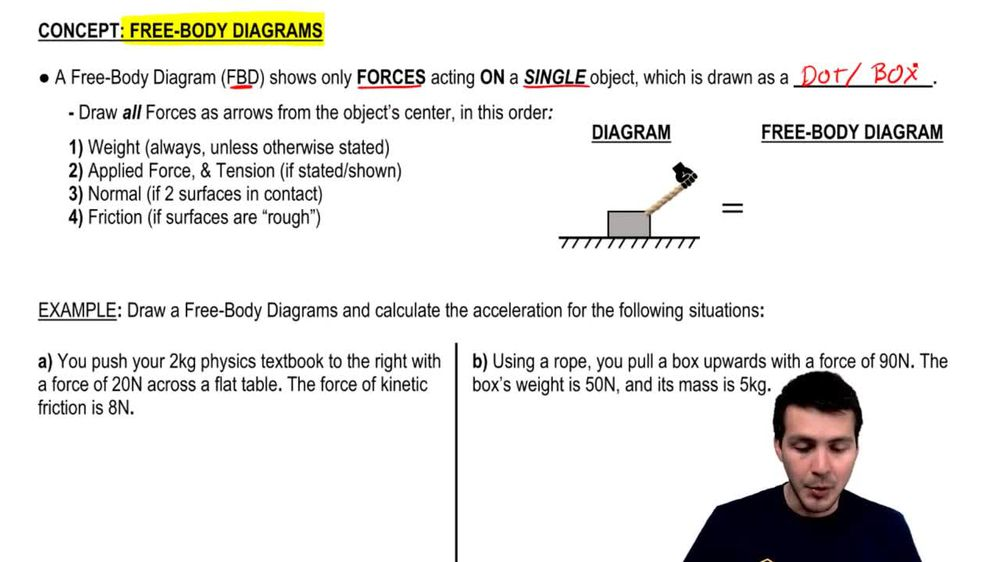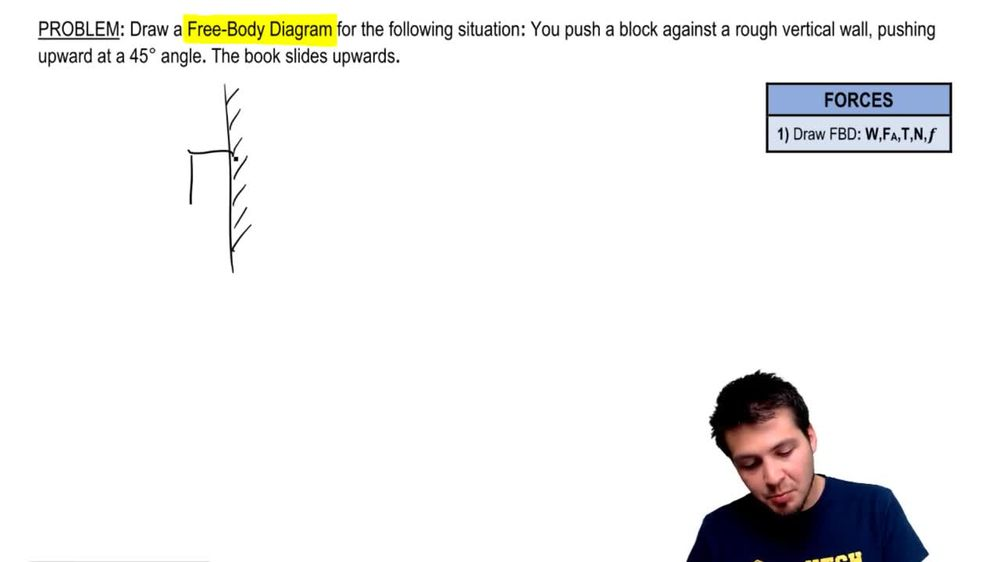Start typing, then use the up and down arrows to select an option from the list.
1. 6. Intro to Forces (Dynamics)2. Types Of Forces & Free Body Diagrams# Drawing a FBD

by Patrick Ford
229 views
0
Hey, guys, gonna practice problem for you. We're gonna go ahead and draw this free body diagram for the following situation. You're taking a block and you're pushing it up against a vertical wall. So I've got the wall like this. We know it's rough. We're gonna take this block, and we're gonna push it up against the wall. And we know the force that we're pushing on it with with this force here makes a 45 degree angle like this. We also know that the book is gonna be sliding upwards, which means the velocity is gonna go up like this. So we have to draw a free body diagram. Remember, this isn't a free body diagram. And instead, we're gonna have to go ahead and look and look for all of the forces here in this particular order to draw our free body diagram. We draw this as a dot or a box like this and we start off with the weight force. Remember that the weight force always acts downwards, right? Unless you're otherwise told towards the Earth's center. So that means that your weight force is going to be down like this. Next we look for any applied forces. This happens whenever you have direct pushes or pulls, and we know we have one here. This is our push at 45 degrees. So we know that this force here is this f we know that acts at degrees. You don't necessarily have to draw the the angle and the diagram. All right, so that's our force. Now we look for tensions, tensions, captains with ropes or strings. And there are no ropes or strings in this problem. So there's no tension, and then now normal. Normal happens when you already have two services that are in contact. So this this block here is being pushed up against this wall. Those two surfaces are touching each other, so there's a normal force that acts perpendicular to the surface. So if the wall is like this, then your normal force is going to be pointing out like this. It doesn't always point upwards, so that's your normal force. So that means we draw in our free body diagrams. So that's our normal. And then finally, when you have frictions, frictions happens. Whatever you have to rough surfaces in contact. So here we have a rough vertical wall. And remember, friction always acts opposing motion. So in our diagram, here we have The velocity of our block was upwards, Which means our friction force is going to oppose that upward velocity. So we know we have a friction force that points downwards like this. That's our free body diagram. Guys. Let me know if you have any question.